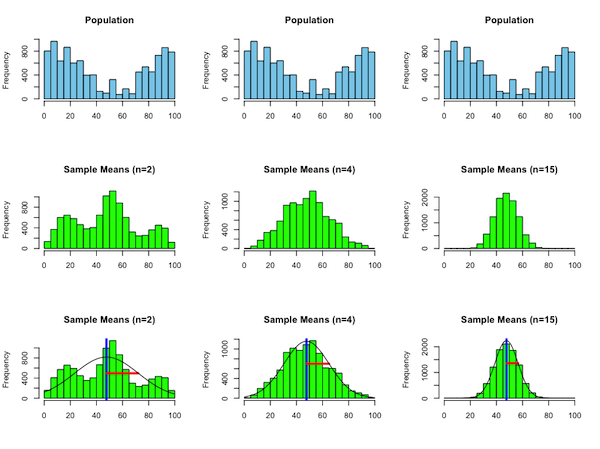## R Project: The Central Limit Theorem

The Central Limit Theorem tells us that the distribution of sample means $\overline{x}$, of samples of size $n$ taken from any given population

1. becomes more "normal" in shape as $n$ increases;
2. has a mean that agrees with the population mean, $\mu$; and
3. has a standard deviation equal to $\sigma/\sqrt{n}$, where $\sigma$ is the standard deviation of the population.

In this project, we will construct a population, and then approximate the distributions of sample means for various sample sizes through repeated sampling, so that we can "see" this theorem in action through a sequence of histograms -- as suggested by the below graphicFirst, we need a population with which to work. Ideally, it will be "far from normal" so that we can see the transition from a non-normal distribution when $n$ is small to a much more normal one when $n$ is large.

For convenience, one can use the following code to construct a population of 10000 values from 1 to 100 that follows a non-normal distribution.

rprobs = sample(1:5,5)
rprobs = rep(16*rprobs,each=20)
rprobs = rprobs + 20*runif(100)
rprobs = rprobs / sum(rprobs)
pop = sample(1:100,size=10000,replace=TRUE,prob=rprobs)


Next, write a function population.hist(pop) that displays a histogram of the population represented by the vector pop that consists of some number of values, each between 1 and 100, inclusive.

• Classes for the histogram should start at $x=0.5$ and be $5$ units wide.
• The bars of the histogram should be colored "skyblue".
• The title of the histogram should be "Population".
• There should be no label on the $x$-axis.

Then, create a second function sample.means(pop,sample.size,n,title,show.overlay) that draws n samples of size sample.size from the population pop, computes their means, and displays a histogram of these means.

• Classes for the histogram should start at $x=0.5$ and be 5 units wide.
• The bars of the histogram should be colored "green".
• The title of this histogram should be taken from the title argument
• There should be no label on the $x$-axis.
• If the show.overlay argument supplied is TRUE, a normal curve centered at the population mean $\mu$ with standard deviation $\sigma/\sqrt{n}$, where $\sigma$ is the population's standard deviation, should be drawn on top of the histogram (scaled vertically, so as to approximate the histogram's shape). Further, a blue vertical line that extends from the bottom to the top of the histogram should mark the position $x = \mu$, and a red horizontal line segment that extends from the aforementioned blue line to the right, with length $\sigma/\sqrt{n}$ should be drawn to indicate the spread of the normal curve drawn. The height of this red line segment should be such that it terminates at a point on the normal curve drawn.
• If the show.overlay argument is FALSE, the normal curve and the blue and red lines mentioned above should not be displayed.
• When the overlay of the normal curve is drawn, the top of the curve should always be visible.

The following observations may help in doing the above:

• The $y$-range for the plot can be specified by the ylim parameter. For example, if ylim=c(0,1000) is used as an argument to the hist() function, $y$-coordinates from 0 to 1000 can be seen in the plot.
• Plots and histograms need not be displayed. One can suppress their display with an argument of plot=FALSE.
• Histograms can be assigned to variables, and a vector of the frequency counts associated with their bars can be accessed, as suggested by the following:
h = hist(v);
bar.counts = h\$counts;

• Finally, the par() function in R can be used to show multiple plots/histograms simultaneously in a grid. Use this, as shown in the below code, to test your work. An image similar to the one shown at the top of this page should result.

par(mfcol=c(3,3))
population.hist(pop)
sample.means(pop,2,1000,"Sample Means (n=2)",FALSE)
sample.means(pop,2,1000,"Sample Means (n=2)",TRUE)
population.hist(pop)
sample.means(pop,4,1000,"Sample Means (n=4)",FALSE)
sample.means(pop,4,1000,"Sample Means (n=4)",TRUE)
population.hist(pop)
sample.means(pop,15,1000,"Sample Means (n=15)",FALSE)
sample.means(pop,15,1000,"Sample Means (n=15)",TRUE)